Ac to Ac Frequency Changer THD Reduction Based on Selective Harmonic Elimination | OMICS International
Journal of Electrical & Electronic Systems

# Ac to Ac Frequency Changer THD Reduction Based on Selective Harmonic Elimination

Ibraheem Mohammed Khaleel*

Department of Computer Communications Engineering, Al-Rafidain University College, Baghdad, Iraq

*Corresponding Author:
Ibraheem Mohammed Khaleel
Department of Computer Communications Engineering
Al- Rafidain University College, Baghdad, Iraq
Tel: 00964-7901278688
E-mail: [email protected]

Received Date: February 03, 2016; Accepted Date: March 03, 2017; Published Date: March 10, 2017

Citation: Khaleel IM (2017) Ac to Ac Frequency Changer THD Reduction Based on Selective Harmonic Elimination. J Electr Electron Syst 6: 214. doi:10.4172/2332-0796.1000214

Copyright: © 2017 Khaleel IM. This is an open-access article distributed under the terms of the Creative Commons Attribution License, which permits unrestricted use, distribution, and reproduction in any medium, provided the original author and source are credited.

Visit for more related articles at Journal of Electrical & Electronic Systems

#### Abstract

AC to AC frequency converters had a challenge to be proposed as a reduction of THD. This challenge due to eliminate the most effective harmonics in frequency spectrum with low cost and no difficulty. However, the challenge is represented by producing new technique for AC to AC frequency changer with lower total harmonic distortion compared with regular AC to AC frequency changer. This proposed work introduces reduction based on eliminate the 3rd predominant harmonic by imposing a waveform with same frequency, amplitude and poly phase of presented harmful harmonic the elimination for the most effective harmonic will cause reduction in THD . The proposed technique gave a significant reduction in THD (about 52%) with respect to regular AC to AC frequency changer with low cost implementation requirements.

#### Keywords

Power electronics; Cycloconverters; Frequency changers; Harmonic reduction techniques; SPWM

#### Introduction

Variable frequency converters became important now a day with every growing demand of industrial applications , in most direct AC to AC frequency changers (step down) high THD has been appeared , the increasing in THD occurs due to higher magnitude of predominant harmonics compared with amplitude of fundamental frequency after dividing the frequency of supply source by (M) where M=2, 3, 4,… etc. , i.e., for frequency changer with M=2 and main supply frequency of 50 Hz the output frequency of frequency changer will be 25 Hz, the frequency spectrum of the output shown only the odd harmonics with different amplitudes and different phases  while the even harmonics had zero magnitude due to even wave symmetry, and harmonic distortion result by the odd harmonics only . One of the important parameters that used to measure the performance in power electronic converters is nth harmonic distortion  caused by the each harmonic which can be estimated using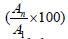where An represent the amplitude of corresponding harmonic while A1 represent the magnitude of fundamental, for divide by two frequency changer described above the nth harmonic distortion resulted by apparent harmonics are subjected in Table 1.

N F (Hz) nth Harmonic distortion 100%
3 75 60.28
5 125 15.12
7 175 6.44
9 225 4.07

Table 1: nth Harmonic distortion for AC to AC frequency changer with M=2.

According to the Table 1, the 3rd harmonic has most effective distortion with respect to other harmonics appeared in frequency spectrum of output waveform for regular frequency changer which is shown in Figure 1a, which frequency spectrum shown in Figure 1b, and the overall distortion result by each apparent harmonics gives THD about 62% .

Figure 1: (a) Output waveform of regular AC to AC frequency converter; (b) Frequency spectrum of regular AC to AC frequency converter.

Harmonics generated by AC to AC frequency changers, increase power system heat losses and power bills of end users. These harmonics related losses reduce system efficiency, cause apparatus overheating, and increase power and air conditioning coasts , according that harmonic currents can have a significant impact on power electronics devises . However many techniques have been investigated in order to reduce THD of frequency changers such as (Comb Filtering, Multi-Phase Comparative Commutation, Half Cycle Omission, PWM Switching) [9-12], the techniques comb filtering, multi-phase comparative commutation depend on estimating the magnitude of predominant harmonic which caused high THD, and trying to reduce the magnitude of these predominant harmonics in order to reduce THD, however the pervious methods depend on magnitude of harmonics and forgetting the phase shift of predominant harmonic effect, also to implement single phase undistorted output waveform, it is necessary to provide at least two balanced distorted output waveforms, or for the PWM technique the output voltage control can be obtained without any external components and PWM minimizes the lower order harmonics, while the higher order harmonics with high nth harmonic distortion has been appeared, which can be eliminated using a filtering techniques, also the value of root mean square voltage of fundamental frequency reduced to significant amount and caused increasing in current that subjected to the load, the previous problem has been appeared in half cycle omission technique, however the problems that described in each technique gives a bad performance for power electronics devices generally and frequency changers specially, according that a new technique to reduce THD of AC to AC frequency changers based on the phase shift of predominant harmonic has been proposed.

#### Proposed Technique

The suggested method represents the effective predominate harmonic of regular AC to AC frequency changer with M=2 in complex polar form as a magnitude and phase shift the elimination process depend on imposing a poly-phase shifted signal with amplitude and frequency equal to magnitude and frequency of harmful third harmonic which can be described in complex form as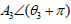, the complex addition can be determined as shown in phasor diagram of in Figure 2.

Which is shows two complex components one of them represent harmful 3rd harmonic and the other represent the imposed poly-phase signal, the resultant of adding these two complex components yields in an elimination of harmful 3rd harmonic, which can mathematically represented:

A3<Æ�3+A3 < (Æ�3+Ð�)=A3 cos (Æ�3)+jA3 sin (Æ�Æ�3)+A3 cos (Æ�3+Ð�)+jA3 sin (Æ�3+Ð�)=A3 cos (Æ�3)+jA3 sin (Æ�3)+A3 cos (Æ�3) cos (Ð�)+A3 sin (Æ�3) sin (Ð�)+j[A3 cos (Æ�3) sin (Ð�)+j+A3 sin (Æ�3) cos (Ð�)]=A3 cos (Æ�3)+jA3 sin (Æ�3) - [A3 cos (Æ�3)+jA3 sin (Æ�3)]=0

However the proposed system depends on generating the poly phase of 3rd harmonic using simple H bridge inverter, and generating distorted waveform using regular AC to AC single phase frequency changer and adding the two singles to-gather as shown in Figure 3.

#### Mathematical Model and Calculation

The proposed technique depend on adding two waveforms of different converters which are differs in frequency, phase, and amplitude one of them represents output of regular AC to AC converter operates with M=2 with frequency can be represented according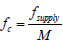which achieve higher THD and the other represents the imposed signal that eliminate the harmful harmonic which is has frequency of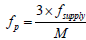. The output of the proposed converter can be represented by the following equation: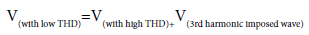(1)

The Fourier transform for both sides according to linearity theorem can be represented as shown in equation (2):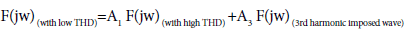(2)

Tï»¿he polar format of Fourier transform estimated, and manipulated at amplitude and phase of each harmonic appears in the frequency spectrum as shown in equation below: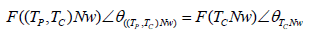(3)

Where (TC) represent the period of distorted wave result by regular AC to AC frequency step down changer, while (TP) represents the period of imposed wave converter, and the frequency spectrum can determined as shown in equation (4):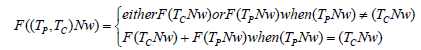(4)

However the output of AC to AC frequency with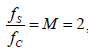where fC represent the output frequency of regular AC to AC frequency changer and fS represent main supply frequency, can be represented using equation (5):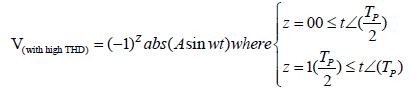(5)

The general form for Fourier series expansion to a periodic waveform can be established using equation (6).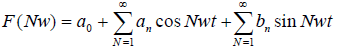(6)

Where,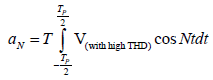(7)

However due to even wave symmetry, the Fourier coefficients (aN) and (aO) are zeros, so that we need evaluate coefficients (bN), which can be determined according (bN) integral, which can be established according to equation (8):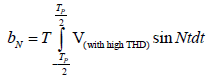(8)

After estimating the above integrals which is outcome the sine Fourier coefficients of frequency spectrum which represented using equation (9) .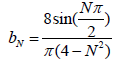(9)

And these coefficients exist for odd integer of N, so that the Fourier series expansion can be established as shown in equation (10):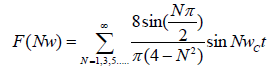(10)

As well as the Fourier series expansion of the imposed signal which generated using unipolar SPWM inverter can be represented in equation (11) .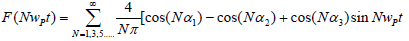(11)

Where α1, α2, α3 represent the switching angles of unipolar PWM.

The amplitude and phase of fundamental and nth harmonics appeared in spectrums according to equations (10 and 11) were denoted in Figure 4, assuming that the imposed waveform with reference signal has poly phase of 3rd harmful harmonic of regular AC to AC frequency changer waveform.

Figure 4: Amplitude and phase calculations for fundamental and nth harmonic for both converters.

The THD of each waveform was estimated and gives 62% for waveform that represent regular AC to AC frequency converter, while for imposed waveform gives THD of 48%, finally resultant spectrums according to equations (10) and (11) separately were determined as shown in Figure 5.

Figure 5: (a) Frequency spectrum of SPWM imposed waveform; (b) Frequency spectrum of regular AC to AC frequency converter.

And the Fourier series of resultant waveform that established according the proposed technique which is described in equation (1), with low THD can be determined using equation (12):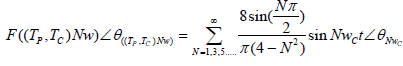(12)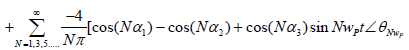Finally the output of the proposed AC to AC frequency changer is estimated according to equation (1) and it is denoted in Figure 6.

The spectrum of output waveform that is denoted in Figure 6, can be estimated using equation (12), the estimated spectrum is shown in Figure 7, which is a combination of the two spectrums described according to equations (10, 11), that is mean the spectrum of regular AC to AC frequency converter and the spectrum of the unipolar SPWM imposed waveform are merged together and the apparent harmonics in the new spectrum have frequencies of (25*N and 75*N) Hz respectively where (N=1, 3, 5...). The first harmonic (N=1 fundamental) has a frequency of 25 Hz with suitable normalized amplitude while 3rd harmonic which has a frequency of 75 Hz was completely eliminated, finally a bank of high frequencies with low amplitude which have nth harmonic distortion does not exceed 12% have been appeared, and the overall THD for proposed converter reduced to 30%.

#### Simulation and Result

The proposed system has been implemented using simulink matlab package, the implementation of this system is divided into two levels: the first one represents a bidirectional bridge converter used to implement regular AC to AC frequency changer which is designed to give 25 Hz high distorted output waveform, while the second level represents rectifier and H bridge circuit used to implement unipolar SPWM inverter which is designed to investigate 75 Hz imposed voltage with phase shift=1800 and amplitude equal to amplitude of 3rd harmonic related by regular AC to AC frequency changer , the addition process of the two voltages resulted from the two converters has been implemented according to superposition theorem across unity linear transformer. The circuit configuration that represents the proposed technique is described in Figure 8.

The output of each level denoted in the proposed Ac to AC frequency converter circuit is described in Figure 9.

The spectrum of resultant simulated output voltage can be represented using FFT algorithm simulator as shown in Figure 10, which is showing a predominant fundamental frequency of 25 Hz with acceptable normalized amplitude, also elimination of harmful third harmonic that appears in frequency spectrum of regular AC to AC frequency changer, the apparent harmonics have frequencies of (25*N) and (N*75) respectively and N=1, 3, 5, 7…, with very low normalized voltages. Finally a bank of high order harmonics with low normalized amplitudes has been appeared without any effect on THD value of the proposed converter.

Due to elimination of 3rd harmonic, the proposed technique gives output waveform with THD about 30% and this value considered to be significant reduction if it is compared with regular AC to AC frequency changer, the reduction in THD enhanced the performance parameters of converter like (efficiency, system cooling requirement cost, overheating current reduction) according to IEEE standard and recommended values [15,16].

#### Conclusion

The proposed system was investigated to give enhanced single phase output waveforms frequency changer if it is compared to the regular AC to AC frequency changer output due to its effective reduction in THD% (about 56% reduction). The proposed system considers being simple and with low cost of implementation, however H bridge inverter used to impose waveform designed to be poly phase and same frequency of 3rd harmful and adding the imposed waveform to output of regular AC to AC frequency converter, the addition process will eliminate 3rd harmful third harmonic, and result a high frequency harmonic components but with low amplitude and doesn’t have significant effect on THD.

#### References

Select your language of interest to view the total content in your interested language

### Article Usage

• Total views: 1777
• [From(publication date):
April-2017 - Jan 21, 2020]
• Breakdown by view type
• HTML page views : 1634Can't read the image? click here to refresh